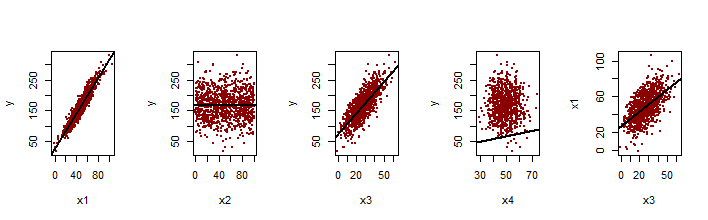After this post.

We build up fictional data around the regression line:

$y = 7.2 + 2.3 \, x_1 + 0.1 \, x_2 + 1.5 \, x_3 + 0.013 \, x_4 + eps$

by using this function:

correlatedValue = function(x, r){
r2 = r**2
ve = 1 - r2
SD = sqrt(ve)
e  = rnorm(length(x), mean = 0, sd = SD)
y  = r * x + e
}

And the following function, which generates four variables (x1, x2, x3 and x4) as well as noise (eps). x1 and x3 are sample from normal distributions; x2 is extracted from a uniform; and x4 from a Poisson.

variables <- function(){
x <- rnorm(1000)
x1 <- 50 + 15 * x
x3 <- 28 + 11 * correlatedValue(x = x, r = 0.6)
x2 <- runif(1000, 0, 100)
x4 <- rpois(1000,50)
eps <- rnorm(1000,5, 7)
y = 7.2 + 2.3 * x1 + 0.001 * x2 + 1.5 * x3 + 0.013 * x4 + eps
dat <- as.data.frame(cbind(y, x1, x2, x3, x4))
c <- as.numeric(coef(lm(y ~ x2 + x3 + x4, dat)))
d <- as.numeric(coef(lm(y ~ x1 + x2 + x3 + x4, dat)))
c(c,d)
}

x1 and x3 are highly influential on y and are correlated with each other, setting the values up to observe OVB. x2 and x4 are less influential.

Here is the plotting of y against x1, x2, x3 and x4, and x1 over x3 with added regression lines:And following is the variance-covariance matrix:

             y           x1           x2         x3          x4
y   1.00000000  0.944410945  0.014421682 0.77571067 -0.01463981
x1  0.94441094  1.000000000 -0.001726526 0.56504020 -0.03562991
x2  0.01442168 -0.001726526  1.000000000 0.03537959  0.02253922
x3  0.77571067  0.565040198  0.035379590 1.00000000  0.02573827
x4 -0.01463981 -0.035629906  0.022539218 0.02573827  1.00000000

Predictably, the regression including all variables shows similar coefficients to the initial equation:

coef(lm(y~.,dat))[2:5]
x1          x2          x3          x4
2.253353226 0.004899445 1.547915198 0.017710038 

Wrapping up, a quick simulation is carried out to obtain the mean of the x3 coefficient in 1,000 simulations WITHOUT including x1 (“coef_x3”) and then WITH x1 (“coef_x3_full”):

coef_x3 <- NULL
coef_x3_full <- NULL
for (i in 1:1000){
coef_x3[i] = variables()
coef_x3_full[i] = variables()
}
mean(coef_x3)
mean(coef_x3_full)

obtaining a coefficient for x3 of 3.383 when x1 is excluded versus a coefficient for x3 of 1.502 when included. So when x1 is included we have an unbiased estimation of the true x3 coefficient (1.5), whereas the estimation is biased when we exclude x1.